## Scope

This article applies for all devices and accessories dealing with very low power signals (antennas, Wifi, 4G, ...).

## Intended Audiance

Public

- Summary of issue

- Solution

- Related Articles

- Troubleshooting

## Prerequisites

Little knowledge on logarithmic calculations and scales.

A logarithm is the exponent to which the number 10 must be raised to reach some  given value. If we are given the number 1000 and asked to find the  logarithm (log), we find that log 1000 = 3 because 10^3 = 1000. Notice  that our logarithm, 3, is the exponent. An important thing to note about  logarithms is that the logarithm of a negative number or of zero does  not exist.

## Summary

Many parameters related to antennas, modems, ...,  are measured in decibels; for instance, gain or loss are often specified in decibels, written as dB.

Or maybe the minimum received power for an antenna system to work is specified as -70 dBm (decibels relative to a milliWatt). Or the transmit power of your cell phone is given as -3 dB.

Why is this? Why don't they just tell you how much power is transmitted in Watts or the gain of an antenna in regular (linear) units?

The decibel system is used when quantities can vary by massive amounts. The Richter scale for earthquakes is also on a scale similar to decibels, because the magnitude of earthquakes can vary from barely perceptible with sophisticated equipment to earthquakes that demolish vast amounts of land.

As an example, lets look at power received by an antenna relative to power transmitted by a tower or Satelitte.

Suppose we have a GPS satellite transmitting 50 Watts of power, with a gain of 2 in the direction of a receive antenna with a gain of 2. Suppose the antennas are separated by 20,000 kilometers (as is the typical orbit of a gps satellite). The GPS satellites operate at a frequency of 1.575 GHz. How much power is received?

Using the Friis Transmission Equation and the fact that wavelength equals c/f, we can calculate the received power to be: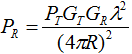Of the 50 Watts transmitted, about 2.3-16 % of that power gets through. Incidentally, this might seem frighteningly small, but yes, antenna systems can operatte with this less power.

Engineers don't like to use linear units when the quantities can vary by such large amounts. Its real tough to picture in your head the difference between 1017 and 1018. To work around this, we use the decibel system.

## Solution

Decibel (dB)

By definition a decibel is a simple logarithmic transformation - units in linear are easily converted via: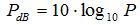Hence, the above quoted value of 1.149-16 Watts is equal to -159.4 decibels, written -159.4 dB.

To give a cemented idea on the conversions, a table of linear to decibel levels is presented.

 Linear Value dB Value 10-10 -100 dB 10-9 -90 dB 10-6 -60 dB 10-3 -30 dB 10-1 -10 dB 0.5 -3 dB 1 0 dB 10 10 dB 100 20 dB 103 30 dB 106 60 dB

Another nice feature of decibels is they make multiplication problems become additions.

Recall the Friis Transmission Formula: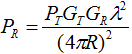(*)

To convert this equation from linear units in Watts to decibels, we take the logarithm of both sides and multiply by 10: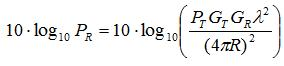A nice property of logarithms is that for two numbers A and B (both positive), the following result is always true:Equation (*) then becomes:Using the definition of decibels, the above equation becomes a simple addition equation in dB:The above representation is easier to work with, which is kind of cool.

dBm

Another common unit is dBm. This means "decibels relative to a milliWatt". In this case, we are talking about power, and the power is just specified in milliWatts instead of Watts.

So:

1 mW = 0  dBm

1 W = 1000 mW = 0 dB = 30 dBm

Or

0 dBm = -30 dB = 0.001 W = 1 mW

The  relationship between the decibels scale and the mW scale can be  estimated using the following rules of thumb:

•         +3 dB will  double the watt value:

(10 mW +  3dB ≈ 20 mW)

•         Likewise,  -3 dB will halve the watt value:

(100 mW -  3dB ≈ 50 mW)

•         +10 dB will  increase the watt value by ten-fold:

(10 mW +  10dB ≈ 100 mW)

•         Conversely,  -10 dB will decrease the watt value to one tenth of that value:

(300 mW -  10dB ≈ 30 mW)

dBi

Another common variation on dB in antenna theory is dBi which means "decibels relative to an isotropic antenna". This just specifies the gain of an antenna relative to the isotropic gain, which is 1. So, the unit of  measurement dBi refers only to the gain of an antenna. The “i” stands  for “isotropic”, which means that the change in power is referenced  against an isotropic radiator.So really nothing changes...:

Gain of 10 dB = Gain of 10 dBi

dBd

This means "decibels of gain relative to a standard half-wave dipole antenna" or "decibels relative to a dipole antenna".

The gain of a half-wave dipole is 2.15 dBi.

So:

Antenna with a gain of 10 dBi = 10 dB = 7.85 dBd

Gain of half-wave diple antenna = 2.15 dBi = 0 dBd

Hence, 7.85 dBd means the peak gain is 7.85 dB higher than a dipole antenna;

this is 10 dB higher than an isotropic antenna.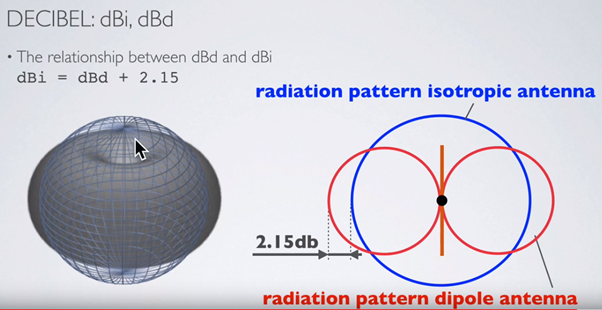## Some formulas

• Gain or Loss (dB) = 10 Log10 P2/P1

P1 = Input power, P2 = Output power

If P2 > P1 : loss (negative number)

if P2 < P2 : gain (positive number)

• Power (dBm) = 10 Log10 (power(mW)/1mW)

or

Power (dBW) = 10 Log10 (power (W)/1W

Note: 0 dBm = 1 mW

Note:  30 dBm = 1 W

Note: +30 dBm = 0 dBW

Note: -30 dBW = 0 dBm

## Related articles

https://help.venntelecom.com/a/solutions/articles/44001930729?lang=en

https://help.venntelecom.com/a/solutions/articles/44001931194?lang=en

https://help.venntelecom.com/a/solutions/articles/44001930999?lang=en